Publicité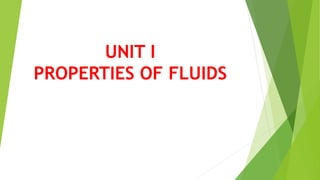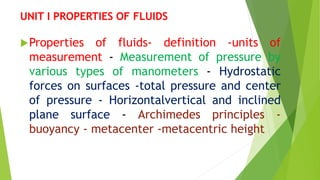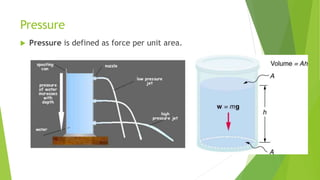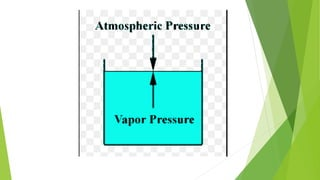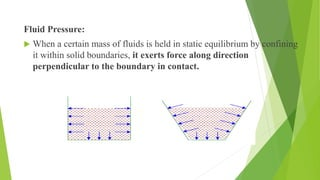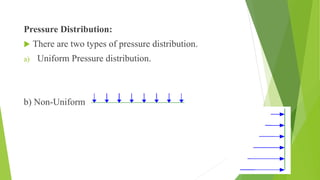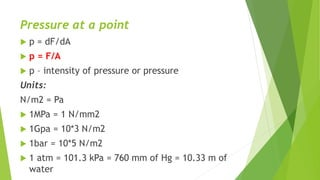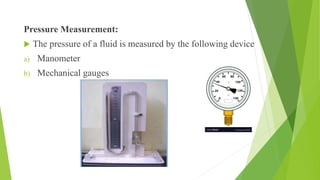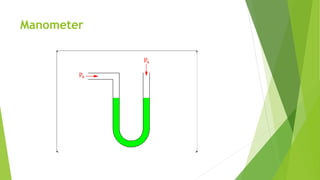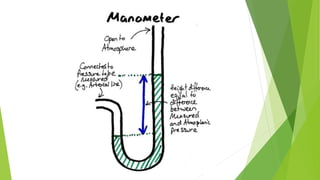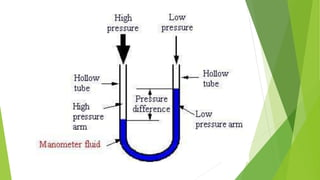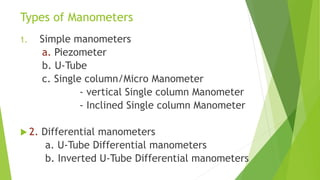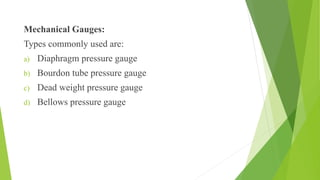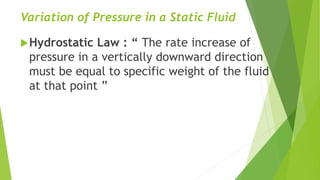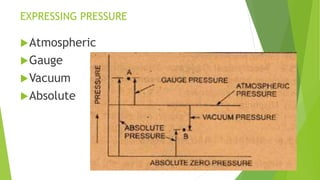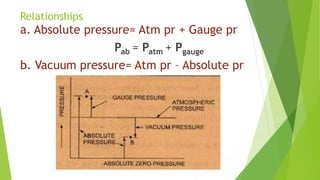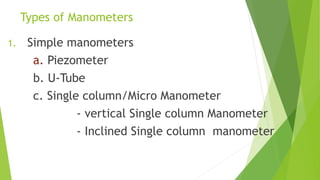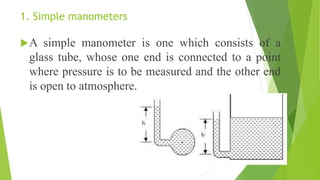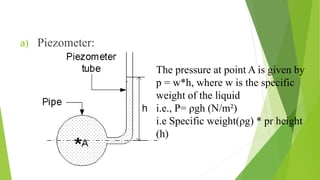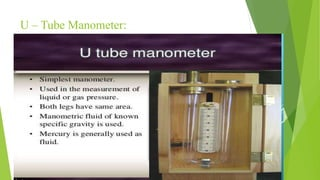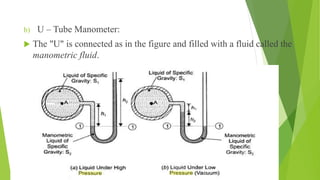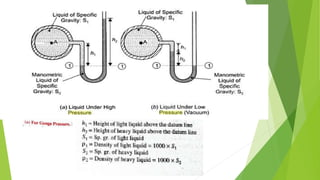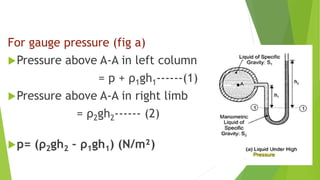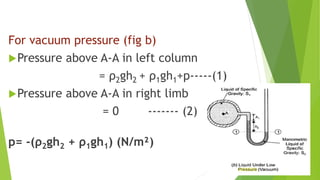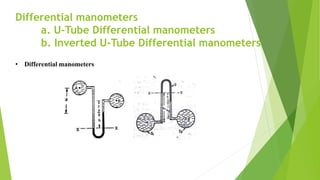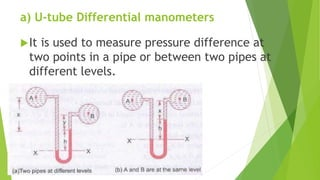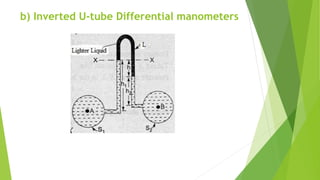1 sur 28
Publicité

### Unit 1.2 mano class

1. UNIT I PROPERTIES OF FLUIDS
2. UNIT I PROPERTIES OF FLUIDS Properties of fluids- definition -units of measurement - Measurement of pressure by various types of manometers - Hydrostatic forces on surfaces -total pressure and center of pressure - Horizontalvertical and inclined plane surface - Archimedes principles - buoyancy - metacenter -metacentric height
3. Pressure  Pressure is defined as force per unit area.
4. Fluid Pressure:  When a certain mass of fluids is held in static equilibrium by confining it within solid boundaries, it exerts force along direction perpendicular to the boundary in contact.
5. Pressure Distribution:  There are two types of pressure distribution. a) Uniform Pressure distribution. b) Non-Uniform Pressure distribution.
6. Pressure at a point  p = dF/dA  p = F/A  p – intensity of pressure or pressure Units: N/m2 = Pa  1MPa = 1 N/mm2  1Gpa = 10*3 N/m2  1bar = 10*5 N/m2  1 atm = 101.3 kPa = 760 mm of Hg = 10.33 m of water
7. Pressure Measurement:  The pressure of a fluid is measured by the following device a) Manometer b) Mechanical gauges
8. Manometer
9. Types of Manometers 1. Simple manometers a. Piezometer b. U-Tube c. Single column/Micro Manometer - vertical Single column Manometer - Inclined Single column Manometer  2. Differential manometers a. U-Tube Differential manometers b. Inverted U-Tube Differential manometers
10. Mechanical Gauges: Types commonly used are: a) Diaphragm pressure gauge b) Bourdon tube pressure gauge c) Dead weight pressure gauge d) Bellows pressure gauge
11. Variation of Pressure in a Static Fluid Hydrostatic Law : “ The rate increase of pressure in a vertically downward direction must be equal to specific weight of the fluid at that point ”
12. EXPRESSING PRESSURE Atmospheric Gauge Vacuum Absolute
13. Relationships a. Absolute pressure= Atm pr + Gauge pr Pab = Patm + Pgauge b. Vacuum pressure= Atm pr – Absolute pr
14. Types of Manometers 1. Simple manometers a. Piezometer b. U-Tube c. Single column/Micro Manometer - vertical Single column Manometer - Inclined Single column manometer
15. Types of Manometers 1. Simple manometers a. Piezometer b. U-Tube c. Single column/Micro Manometer - vertical Single column Manometer - Inclined Single column Manometer  2. Differential manometers a. U-Tube Differential manometers b. Inverted U-Tube Differential manometers
16. 1. Simple manometers A simple manometer is one which consists of a glass tube, whose one end is connected to a point where pressure is to be measured and the other end is open to atmosphere.
17. a) Piezometer: The pressure at point A is given by p = w*h, where w is the specific weight of the liquid i.e., P= ρgh (N/m²) i.e Specific weight(ρg) * pr height (h)
18. U – Tube Manometer:
19. b) U – Tube Manometer:  The "U" is connected as in the figure and filled with a fluid called the manometric fluid.
20. For gauge pressure (fig a) Pressure above A-A in left column = p + ρ1gh1------(1) Pressure above A-A in right limb = ρ2gh2------ (2) p= (ρ2gh2 – ρ1gh1) (N/m²)
21. For vacuum pressure (fig b) Pressure above A-A in left column = ρ2gh2 + ρ1gh1+p-----(1) Pressure above A-A in right limb = 0 ------- (2) p= -(ρ2gh2 + ρ1gh1) (N/m²)
22. Differential manometers a. U-Tube Differential manometers b. Inverted U-Tube Differential manometers • Differential manometers
23. a) U-tube Differential manometers It is used to measure pressure difference at two points in a pipe or between two pipes at different levels.
24. b) Inverted U-tube Differential manometers
Publicité